# JAC Class 9 Science Important Questions Chapter 9 Force and Laws of Motion

## JAC Board Class 9th Science Important Questions Chapter 9 Force and Laws of Motion

Multiple Choice Questions

Question 1.
The SI unit of force is
(a) kg m/s
(b) newton
(c) dyne
(d) kg wt
(b) newton

Question 2.
The combined effect of mass and velocity is taken into account by a physical quantity called
(a) torque
(b) momentum
(c) moment
(d) force
(b) momentum

Question 3.
Quantitative definition of force is given by
(a) Newton’s first law of motion
(b) Newton’s second law of motion
(c) Newton’s third law of motion
(d) Newton’s law of gravitation
(b) Newton’s second law of motion

Question 4.
Momentum gives a measure of
(a) mass
(b) weight
(c) velocity
(d) motion
(d) motion

Question 5.
An athlete runs some distance before taking a long jump because
(a) he gains energy to take himself through long distance
(b) it helps him to apply a larger force
(c) by running, action and reaction forces increase
(d) by running, the athlete gives himself larger inertia of motion
(d) by running, the athlete gives himself larger inertia of motion

Question 6.
Rocket works on the principle of conservation of
(a) velocity
(b) energy
(c) linear momentum
(d) mass
(c) linear momentum

Question 7.
The rate of change of momentum of an object is proportional to the
(a) mass of the body
(b) velocity of the body
(c) net force applied on the body
(d) none of these
(c) net force applied on the bodyQuestion 8.
A force of 50 N moves a body. Which of the following is correct?
(a) Frictional force exerted on the body is less than 50N
(b) Frictional force exerted on the body is more than 50N
(c) None of these
(d) Both (a) and (b)
(a) Frictional force exerted on the body is less than 50N

Question 9.
If a football and a stone have same mass, then both will have
(a) same inertia
(b) same momentum
(c) different inertia
(d) different momentum
(a) same inertia

Question 10.
The rate of change of momentum w. r. t time is measured in
(a) kg m
(b) kg
(c) kg ms-1
(d) kg ms-1
(d) kg ms-1

Question 11.
A block of mass M is pulled with a force F along a smooth horizontal surface with a rope of mass m. The acceleration of the block will be
(a) F/M
(b) F/m
(c) F/(M + m)
(d)F/(M – m)
(c) F/(M + m)

Question 12.
Action and reaction forces act on two
(a) different objects
(b) same objects
(c) either (a) or (b)
(d) none of these
(a) different objects

Question 13.
A man is standing in a boat in still water. If he tries to walk towards the shore, the boat will
(a) move away from the shore
(b) remain stationary
(c) sink
(d) move towards the shore
(a) move away from the shore

Question 14.
When an object undergoes acceleration
(a) its speed always increases
(b) its velocity always increases
(c) it always falls towards the earth
(d) a force always acts on it
(d) a force always acts on it

Question 15.
On applying a constant force to a body, it moves with uniform
(a) momentum
(b) speed
(c) acceleration
(d) velocity
(c) acceleration

Analysing & Evaluating Questions

Question 16.
A goalkeeper in the game of football pulls his hands backwards after holding the ball shot at the goal. This enables the goal keeper to
(a) exert larger force on the ball
(b) reduce the force exerted by the ball on hands
(c) increase the rate of change of momentum
(d) decrease the rate of change of momentum
(b) reduce the force exerted by the ball on hands

Question 17.
A passenger in a moving train tosses a coin which falls behind him. It means that motion of the train is
(a) accelerated
(b) uniform
(c) retarded
(d) along circular tracks
(a) acceleratedQuestion 18.
An object of mass 2kg is sliding with a constant velocity of 4 ms-1 on a frictionless horizontal table. The force required to keep the object moving with the same velocity is
(a) 32 N
(b) 0 N
(c) 2 N
(d) 8 N
(b) 0 N

Assertion Reason Questions

Directions: In the following questions, the Assertions and the Reasons have been put forward Read the statements carefully and choose the correct alternative from the following:
(A) Both the assertion and the reason are correct and the reason is the correct explanation of the assertion.
(B) The assertion and the reason are correct but the reason is not the correct explanation of the assertion.
(C) The assertion is true but the reason is false.
(D) Both statements are false.
1. Assertion: A brick has more inertia than a hollow wooden block of the same shape and size.
Reason: Heavier the body, more is the inertia.
(A) Both the assertion and the reason are correct and the reason is the correct explanation of the assertion.

2. Assertion: A body falling towards the earth also pulls the earth towards itself.
Reason: The forces always occur in pairs.
(A) Both the assertion and the reason are correct and the reason is the correct explanation of the assertion.

3. Assertion: When a bus starts, the person inside it falls forward.
Reason: The bus pushes the person forward.
(D) Both statements are false.

4. Assertion: The third law of motion defines acceleration.
Reason: The third law of motion states that the net force experienced by a body is proportional to the rate of change in momentum of the body.
(D) Both statements are false.

5. Assertion: Action and reaction forces are always equal and opposite.
Reason: Action and reaction forces cancel each other.
(C) The assertion is true but the reason is false.

Very Short Answer Type Questions

Question 1.
Define force.
Force may be defined as a push or a pull which changes or tends to change the state of rest or of uniform motion or direction of motion of a body.

Question 2.
Define one Newton.
One newton is the force which produces an acceleration of m/s2 in an object of mass 1kg.
1N = 1kg m/s2

Question 3.
State the various effects of force.
A force applied on an object can do five things:
(a) It can change the speed of the object.
(b) It can change the direction of motion of the object.
(c) It can change the shape and size of the object.
(d) It can set an object at rest in motion.
(e) It can bring a moving object to rest.

Question 4.
Define resultant force.
The resultant force or resultant of several forces acting simultaneously on a body is that single force which produces the same effect on a body as all these forces produce together.Question 5.
What is frictional force?
The force that always opposes the motion of an object is called force of friction.

Question 6.
What is inertia?
The natural tendency of an object to resist a change in their state of rest or of uniform motion along a straight line is called inertia.

Question 7.
State Newton’s first law of motion.
An object remains in its state of rest or of uniform motion in a straight line unless acted upon by an external unbalanced force.

Question 8.
Place a water – filled tumbler on a tray. Hold the tray and turn around as fast as you can. We observe that the water spills. Why?
The water spills when the tray turns around fastly, because initially, the water filled in the tumbler placed on the tray was in a state of rest. While turning, we apply force on the tray which starts moving but the water remains at rest due to its inertia, and hence, it spills out of the tumbler.

Question 9.
State Newton’s second law of motion.
The rate of change of momentum of an object is proportional to the applied unbalanced force in the direction of the force.

Question 10.
State Newton’s third law of motion.
To every action, there is an equal and opposite reaction and they act on two different bodies.

Question 11.
Why are shockers used in cars, scooters and motorcycles?
Due to the shockers, the time interval of the jerk increases. As the rate of change of momentum will be smaller, comparatively less force acts on the passengers during the jerks.

Question 12.
Why is glass or chinaware packed with straw?
The straw paper between the chinaware increases the time of experiencing the jerk during transportation. Hence, they strike against each other with a less force and are less likely to be damaged.

Question 13.
A bird hit the windscreen of a fast moving car and fell on the bonnet. Which of the two, the car or the bird, suffers greater change in momentum?
By the law of conservation of momentum, both the car and the bird suffered equal and opposite change in momentum.

Question 14.
What decides the rate of change of momentum of an object?
The rate of change of momentum of an object is proportional to the applied unbalanced force in the direction of force.

Question 15.
The diagram shows a moving truck. Forces A, B, C and D are acting on the truck. Name the type of each of these forces acting on the truck.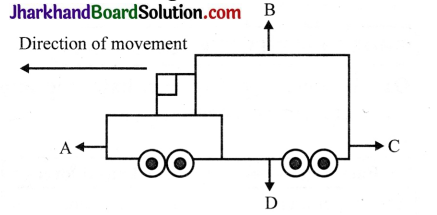The forces A, B, C and D acting on the truck are:
A driving force
B normal reaction
C frictional force
D weight gravitational force.

Analysing & Evaluating Questions

Question 16.
Two identical bullets are fired, one by a light rifle and another by a heavy rifle, with the same force. Which rifle will hurt the shoulder more and why?
The velocity of recoil will be higher for ligher rifle. So lighter rifle will hurt the shoulder more.

Question 17.
When a fast moving carrom striker hits the carrom coin at the bottom of a pile, ¡t moves out without disturbing
the pile. Which kind of inertia of the pile is responsible for it?
Inertia of rest of the pile is responsible for it.

Question 18.
There are three solid balls made up of aluminium, steel and wood, of the same shape and same volume. Which of them would have the highest inertia?
Steel ball will have the highest inertia This is because the density of steel is higher than aluminium and wood As a result, the steel ball will have the highest mass and hence highest inertia

Short Answer Type Questions

Question 1.
Differentiate between balanced and unbalanced forces.

 Balanced forces Balanced forces 1. The resultant of balanced forces is zero. 1. The resultant of balanced forces is zero. 2. These forces donot produce anyacceleration in the body. 2. These forces donot produce anyacceleration in the body. 3. Example: A book kept on the table (the downward force of gravity on the book is balanced by the normal reaction by the table on the book in upward direction). 3. Example: When the book that is kept on the table is pushed to the left side (the book moves as the push towards left is greater than the frictional force acting towards right).

Question 2.
Give examples to show that balanced forces can change the shape of an object.
Consider a spring attached to a rigid support at one of its ends, as shown in the figure.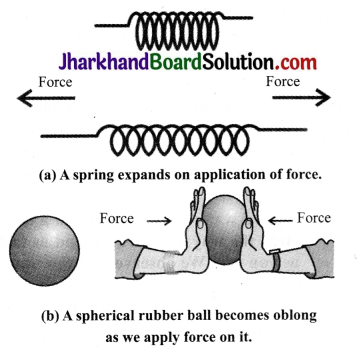If we pull the free ends of the spring, it gets elongated Thus, on applying force, a spring expands. Similarly, if we hold a rubber ball between our palms and push the two palms against each other, we find that the ball is no longer round but oblong. The force exerted on the ball changes its shape, as shown in the figure. In both cases, the two forces, being equal and opposite, balance each other but change the shape of the object.

Question 3.
A football player kicks the ball which travels in the air for a while and lands on the ground where the ball travels on the ground for a short distance and then stops. What may be the reason behind it?
The reason is frictional force. There is always a contact between the ball and the ground The opposing force always acts against the motion of the ball, thereby stopping the ball.Question 4.
A table is moved across the floor with a constant velocity where the horizontal force acting on it is 400N. What will be the frictional force that will be acting on the table?
According to Newton’s third law of motion, for every action there will be an equal and opposite reaction. The net force acting on the table is zero because the table moves with constant velocity in forward direction. Hence, an equal amount of frictional force must act in opposite direction as that of the table, i.e., F = – 400N.

Question 5.
When the car, we are moving in, makes a sharp turn at a very high speed, we tend to get thrown to one side. Explain the statement.
The reason behind this is the law of inertia While moving in a car along a straight line, we tend to maintain our state of motion. But when the car takes a sharp turn, our bodies resist the change in direction and are thrown to the opposite side due to inertia.

Question 6.
Athletes always have a special posture by resting their right foot on a solid support, why?
During the race, athletes have to run the heats and they rest their right foot on the solid support before the start, so that this support can give them a lot of force during the start of the race. They push the support backwards and get an equal and opposite forward push to get a very good start.

Question 7.
The safety belts in the car help in preventing accidents. Justify the statement.
Yes, safety belts help in preventing accidents. When a car is moving with a high speed, our body tends to be in movement due to inertia of motion in the forward direction. So when there is a sudden  collision, serious injuries can happen. However, seat belts exert a force on our body to slow down the forward motion and hence prevent injuries.

Question 8.
A karate player breaks the pile of tiles with a single blow. Give reason.
A karate player strikes the pile of tiles by applying a very large velocity. The overall momentum of fast moving hand is reduced to zero in a very short interval. So this increases the rate of change of momentum which increases the force on the tiles and they eventually break.

Question 9.
Athletes are made to fall on a sand bed while performing a high jump. Give reason.
During the high jump event, an athlete is made to fall on the sand bed because it increases the time to attain the rest
position. We know that, F = $$\frac{m(v-u)}{t}$$
So, force and time are inversely proportional to each other. If there is an increase in time, there is a decrease in rate of change of momentum and therefore the force or the impact is reduced This prevents injuries to the feet of athlete.

Question 10.
Explain the law of conservation of momentum.
According to the law of conservation of momentum, the total momentum of the objects before collision is equal to the total momentum after collision, provided there is no external unbalanced force acting on them.Question 11.
A bullet of mass 20g moving with a speed of 500 ms-2 strikes a wooden block of mass 1kg and gets embedded in it. Find the speed with which the block moves along with the bullet.
Let the final velocity of the block along with the bullet embedded in it be v.
For bullet, m1 = 20g = 0.02 kg,
U1 = 500 m/s, v1 = v
For block, m1 = 1kg, u2= 0, v2 = v
According to the law of conservation of momentum,
Total momentum before collision = Total momentum after collision
m1u1 + m2u2 = m1v1 + m2v2
m1u1 + m2u2= (m1 + m2) v
v = $$\frac{m_{1} u_{1}+m_{2} u_{2}}{m_{1}+m_{2}}$$
= $$\frac{(0.02 \times 500)+(1 \times 0)}{(0.02+1)}$$
=$$\frac{10}{1.02}$$ = $$\frac{1000}{102}$$
= 9.8 m/s.

Analysing & Evaluating Questions

Question 12.
Two balls of the same size but of different materials, rubber and iron, are kept on the smooth floor of a moving train. The brakes are applied suddenly to stop the train. Will the balls start rolling? If so, in which direction? Will they move with the same speed?

The balls will start rolling in the forward direction. No. The two balls will move with different speed This is because the inertia of the two balls is not the same.

Question 13.
Two forces F1 = 40N and F2 = 60N are acting on an object as shown in the figure.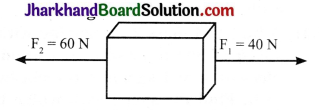1. What is the net force acting on the object?
2. What is the direction of the net force acting on the object?
3. How much extra force is acting on the object if the object is not moving due to the application of these two forces? Name that force. Where does that force act and what is the direction of that force?

1. Net force on the object = 60N – 40N = 20N
2. Net force of 20N acts in the direction of F2 (i.e., towards left)
3. A force equal to 20N is acting on the object.
This force is the frictional force. This force acts in the direction of F1

Long Answer Type Questions

Question 1.
Describe Galileo’s experiments with inclined planes and state their conclusion.
By observing the motion of objects on inclined planes, Galileo deduced that objects move with constant speed when no force acts on them.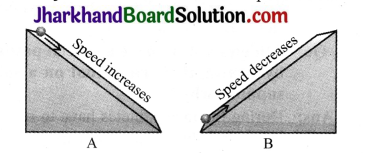1. In the first experiment, Galileo studied the motion of marbles on an inclined plane. He observed that when a marble rolls down an inclined plane, its velocity increases, as shown in the figure A.
2. Here the marble falls under the unbalanced force of gravity. The velocity of the marble decreases when it rolls up the inclined plane (against the force of gravity), as shown in the figure B.
3. From these observations, Galileo argued that the velocity of a marble rolling on a flat horizontal surface should remain constant. To test his idea, Galileo used double inclined planes.
4. He observed that when the inclinations of the planes on both sides were equal, the marble rolled down from one plane from a certain height will climb up to the same height on the other plane.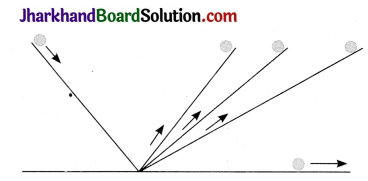side plane were gradually decreased, the marble would travel larger and larger distances to reach the same height.
5. Ultimately, if the right side plane were made horizontal, the marble would continue to travel forever to reach the same height from which it was released No unbalanced force acts on the marble in this case.
6. The above experiment suggests that an unbalanced force (external force) is required to change the motion of an object while no unbalanced force is needed to keep an object moving with a constant velocity.
7. In actual practice, the bodies stop due to the force of friction which always acts opposite to the direction of motion.

Question 2.
Mathematically explain the concept of conservation of momentum during collision between two bodies.
Let us consider two bodies, viz., A and B, moving with initial velocities uA and uB respectively, such that uA > uB.
Let mass of body A be mA and that of B be mB.
Before collision
For body A, momentum = mAuA
For bodv B. momentum = mBuB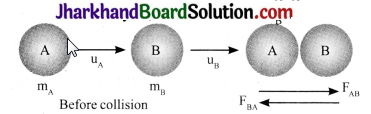After collision, the bodies A and B move with velocities, say, vA and vB respectively. Let the collision occur for a time, say, t.
After collision
For body A, momentum = mAvA
For body B, momentum = mBvB
The rate of change of momentum for the body A = $$\frac{m_{A}\left(v_{A}-u_{A}\right)}{t}$$
The rate of change of momentum for the body A = $$\frac{m_{B}\left(v_{B}-u_{B}\right)}{t}$$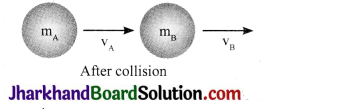According to Newton’s third law of motion, The force FAB exerted by ball A on ball B and the force FBA exerted by the ball B on ball A must be equal and opposite to each other. Therefore
$$\left\{\frac{m_{A}\left(v_{A}-u_{A}\right)}{t}=-\left(\frac{m_{B}\left(v_{B}-u_{B}\right)}{t}\right)\right\}$$
mAuA + mBuB= mAvA + mBvB
Here, (mAuA + mBuA) is the total momentum of the two bodies, A and B, before collision and (mAvA+ mBvB) is the total momentum of the two bodies, A and B, after collision. So, the total momentum of the two bodies remains constant during collision, when no external force acts on them.Question 3.
Give some examples of Newton’s third law of motion.
Newton’s third law of motion states that: For every action, there is always an equal and opposite reaction acting on two different bodies.
Examples:

1. The person who is swimming, pushes the water backward (action), while water pushes the person forward (reaction).
2. When a bullet is fired from a gun, the gun exerts a force on the bullet (action), the bullet exerts an equal and opposite force on the gun (reaction),
3. When a rower jumps out of the boat in the forward direction (action), the boat moves backward (reaction).

Question 4.
Define the term ‘inertia’. Explain its types and state some of its examples in daily life.

1. The tendency of a body to resist change in its state of rest or of uniform motion is called inertia.
• Inertia of rest: The ability of a body to resist any change in its state of rest.
• Inertia of motion: The ability of a body to resist any change in its state of uniform motion.
• Inertia of direction: The ability of a body to resist any change in its direction of motion.
2. Examples of application of inertia in our day to day life are as follows:
• When a carpet is beaten with a stick, the dust falls down.
• When the car applies a sudden brake, we tend to fall forward.
• When a bus suddenly takes a turn, we fall in the direction opposite to the turn.

Question 5.
Mathematically explain the concept of Newton’s second law of motion and also explain its importance in sports.
Newton’s second law of motion: The rate of change of momentum of an object is directly proportional to the applied unbalanced force and it acts in the direction of force.

Example: Let us consider an object of mass (m), moving along a straight line with an initial velocity (u). Suppose, it attains a final velocity (v) in time (t).
Initial momentum of the object
= p1 = mu
Final momentum of the object
= p2 = mv
The change in momentum
= p2 – p1 = (mv) – (mu) = m (v – u)
The rate of change in momentum
= $$\frac{\mathrm{m}(\mathrm{v}-\mathrm{u})}{\mathrm{t}}$$
Therefore,
The applied force, F is
F ∝ $$\frac{\mathrm{m}(\mathrm{v}-\mathrm{u})}{\mathrm{t}}$$
F = $$\frac{\mathrm{mk}(\mathrm{v}-\mathrm{u})}{\mathrm{t}}$$ …………..(i)
(where k is proportionality constant)
From the first equation of motion,
v = u + at
Substituting (ii) in (i),
So, F = kma
The unit of force is so chosen that the value of k becomes one.
Hence, F = kma or
F = ma
The second law is related to sports in the following examples:
(a) In a cricket match, a fielder who is trying to attempt a catch, generally pulls his hands along the moving ball because it consumes time to bring the momentum of the ball to zero. This decreases the rate change of mometum, and hence the force. So, when the time is increased, the overall force reduces, thus saving the hand from getting injured.

(b) In a high jump athletic event, the athletes are made to fall on a sand bed to increase the time of the athlete’s fall to stop after making the jump.
This decreases the rate of change of momentum and hence the force,

(c) A karate expert breaks a slab of ice with a single blow as he moves his hand very fast and then stops it in a very short time. Since the rate of change of momentum increases, the force also increases and the ice slab breaks.

Analysing & Evaluating Questions

Question 6.
A test tube containing little water is corked and suspended from two strings in a slanting position. Afterwards, the test tube is heated Answer the following: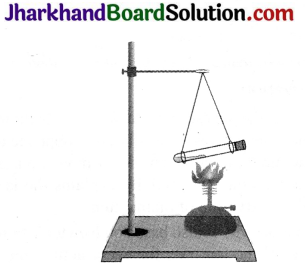1. What happens to the cork?
2. In which direction does the test tube move?
3. Compare the velocities of the cork and of the recoiling test tube.

1. On heating, the water inside the test tube vaporises and the cork is blown out.
2. The test tube moves in the direction opposite to the direction in which the cork has blown out.
3. Cork blows out with greater velocity than the recoiling test tube.

Activity 1

• Make a pile of similar carom coins on a table, as shown in the figure.
• Attempt a sharp and strong horizontal hit at the bottom of the pile using another carom coin or striker.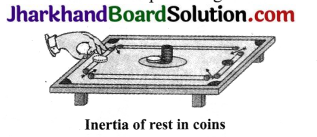Observations

• If the hit is strong enough the bottom coin moves out quickly.
• Once the lowermost coin is removed, the inertia of the other coins makes them fall vertically on the table.

Activity 2

• Set a five – rupee coin on a stiff card covering an empty glass tumbler standing on a table as shown in the figure.
• Give the card a sharp horizontal flick with a finger.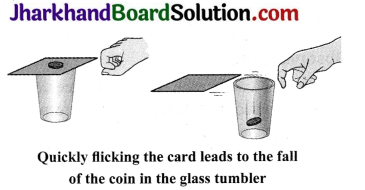Observations

• If we do it fast, the card shoots away, allowing the coin to fall vertically into the glass tumbler due to its inertia.
• The inertia of the coin tries to maintain its state of rest even when the card is flown away.
• The force applied on the card due to flicking changes the inertia of the card but the coin resists this change and stays at rest, i.e, due to the inertia and gravity, the coin falls down into the tumbler.Activity 3

• Request two children to stand on two separate carts as shown in the figure.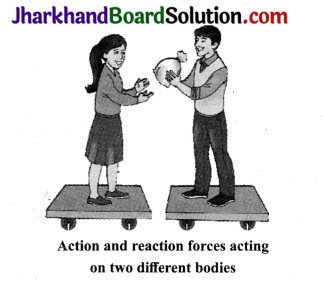• Give them a bag full of sand or some other heavy object.
• Ask them to play a game of catch with the bag and observe.
• You can paint a white line on cartwheels to observe the motion of the two carts when the children throw the bag towards each other.

Observations

• In this case, each of them receives an instantaneous reaction as a result of throwing the sand bag.
• This activity explains Newton’s third law of motion, i.e., a force is exerted in forward direction in throwing the bag full of sand and the person who is throwing it, gets pushed backward.

Activity 4

• Take a big rubber balloon and inflate it fully.
• Tie its neck using a thread Also, using adhesive tape, fix a straw on the surface of this balloon.
• Pass a thread through the straw and hold one end of the thread in your hand or fix it on the wall.
• Ask your friend to hold the other end of the thread or fix it on a wall at some distance.
• The arrangement is shown in the figure.
• Now, remove the thread tied on the neck of the balloon and let the air escape from the mouth of the balloon.
• Observe the direction in which the straw moves.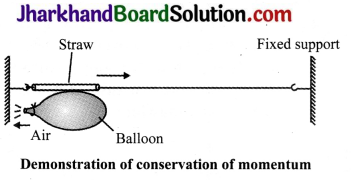Observations.

• When the air escapes out from the balloon, the straw moves in the direction opposite to the direction in which the air moved out of the balloon. This activity explains the law of conservation of momentum.
• This activity is also based on Newton’s third law of motion, i.e., for every action, there is always an equal and opposite reaction between two bodies.

Value Based Questions

Question 1.
Class IX students were playing cricket with cork ball in the school campus. Abhishek, senior student, told them about the accidents that can occur due to cork ball in the campus and also advised them to bring a soft cosco ball to play the game.
1. Why is it safe to play with a soft ball and not with a hard cork ball?
2. A player pulls his hands backwards while catching the ball shot at high speed Why?
3. What value of Abhishek is seen in this act?

1. The soft ball has less inertia as compared to the heavy ball and it would not hurt the players.
2. By pulling the hand backwards, it reduces the force exerted by the ball
on the hand.
3. Abhishek showed the value of being responsible and helpful by nature.

Question 2.
Rahul saw his karate expert friend breaking a brick. He tried to break the brick but his friend stopped him from doing so and told him that it would hurt him as one needs a lot of practice in doing so.
1. How can a karate expert break the brick without any injury to his hand?
2. What is Newton’s third law of motion?
3. What value of Rahul’s friend is seen in the above case?